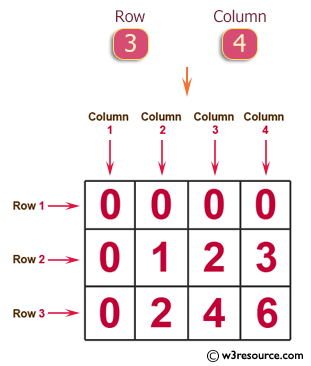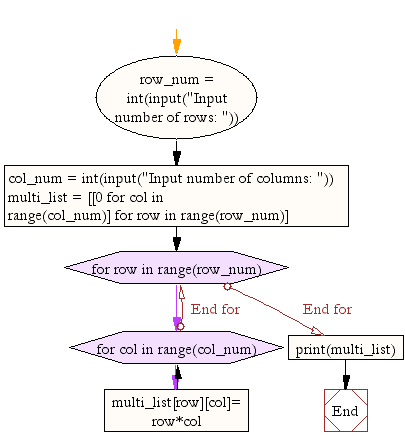﻿ Python Exercise: Generates a two-dimensional array - w3resource# Python Exercise: Generates a two-dimensional array

## Python Conditional: Exercise-11 with Solution

Write a Python program which takes two digits m (row) and n (column) as input and generates a two-dimensional array. The element value in the i-th row and j-th column of the array should be i*j.
Note :
i = 0,1.., m-1
j = 0,1, n-1.

Pictorial Presentation:Sample Solution:

Python Code :

``````row_num = int(input("Input number of rows: "))
col_num = int(input("Input number of columns: "))
multi_list = [[0 for col in range(col_num)] for row in range(row_num)]

for row in range(row_num):
for col in range(col_num):
multi_list[row][col]= row*col

print(multi_list)
```
```

Sample Output:

```Input number of rows: 3
Input number of columns: 4
[[0, 0, 0, 0], [0, 1, 2, 3], [0, 2, 4, 6]]
```

Flowchart:## Visualize Python code execution:

The following tool visualize what the computer is doing step-by-step as it executes the said program:

Python Code Editor :

Have another way to solve this solution? Contribute your code (and comments) through Disqus.

What is the difficulty level of this exercise?

Test your Python skills with w3resource's quiz

﻿

## Python: Tips of the Day

Returns a list with n elements removed from the beginning

Example:

```def tips_take(itr, n = 1):
return itr[:n]
print(tips_take([1, 2, 3], 5))
print(tips_take([1, 2, 3], 0))
```

Output:

```[1, 2, 3]
[]
```